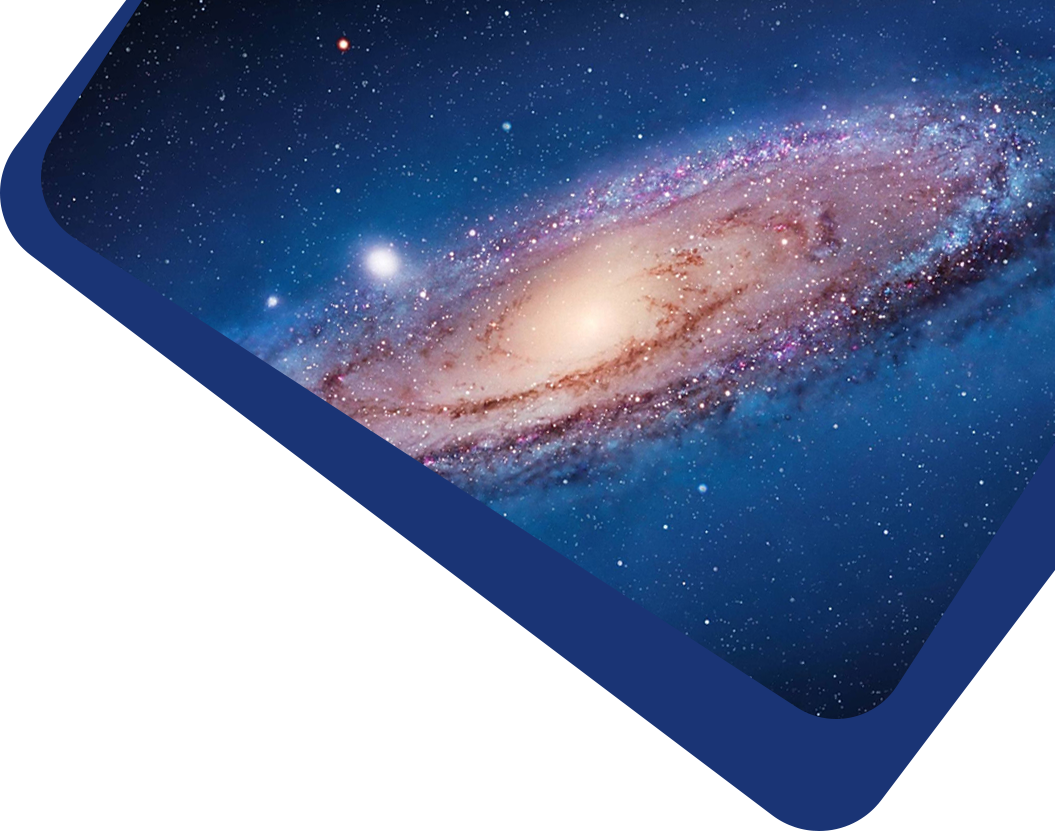Vol 22, No 1

## Spot and Facula Activity Variations of the Eccentric Detached Eclipsing Binary KIC 8098300 Based on the Time-series Orbital Solutions

#### Hu-Biao Niu, Jian-Ning Fu, Jia-Xin Wang, Chun-Qian Li

Abstract

Abstract The LAMOST spectra and Kepler light curves are combined to investigate the detached eclipsing binary KIC 8098300, which shows the O’Connell effect caused by spot/facula modulation. The radial velocity (RV) measurements are derived by using the tomographic spectra disentangling technology. The mass ratio $$q = K_1/K_2 = 0.812 \pm 0.007$$, and the orbital semimajor axis $$a \sin i = 14.984\pm 0.048 R_{\odot}$$ are obtained by fitting the RV curves. We optimize the binary model concerning the spot/facula activity with the code PHOEBE and obtain precise parameters of the orbit including the eccentricity $$e = 0.0217 \pm 0.0008$$, the inclination $$i = 87^{\circ}.71 \pm 0^{\circ}.04$$, and the angle of periastron $$\omega = 284^{\circ}.1 \pm 0^{\circ}.5$$. The masses and radii of the primary and secondary star are determined as $$M_1 = 1.3467 \pm 0.0001 M_{\odot}$$, $$R_1 = 1.569 \pm 0.003 R_{\odot}$$, and $$M_2 = 1.0940 \pm 0.0001 M_{\odot}$$, $$R_2 = 1.078 \pm 0.002 R_{\odot}$$, respectively. The ratio of temperatures of the two component stars is $$r_{\rm teff} = 0.924 \pm 0.001$$. We also obtain the periastron precession speed of 0.000024 ± 0.000001 day cycle−1. The residuals of out-of-eclipse are analyzed using the auto-correlation function and the discrete Fourier transform. The spot/ facula activity is relatively weaker, but the lifetime is longer than that of most single main sequence stars in the same temperature range. The average rotation period of the spots $$P_{\rm rot} = 4.32$$ days is slightly longer than the orbital period, which may be caused by either the migration of spots/faculae along the longitude or the latitudinal differential rotation. The activity may be spot-dominated for the secondary star and facula-dominated for the primary star. The method of this work can be used to analyze more eclipsing binaries with the O’Connell effect in the Kepler field to obtain the precise parameters and investigate the difference of spot behavior between binaries and single stars. It is helpful for a deeper understanding of the stellar magnetic activity and dynamo theory.

Keywords

Keywords binaries: eclipsing – (stars:) starspots – stars: individual

Full Text
Refbacks

• There are currently no refbacks.